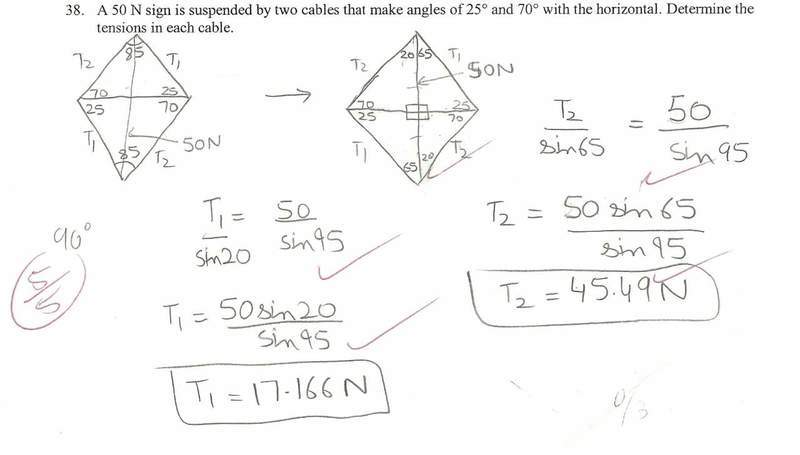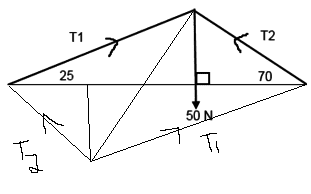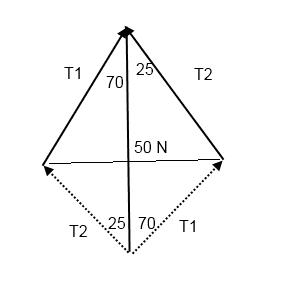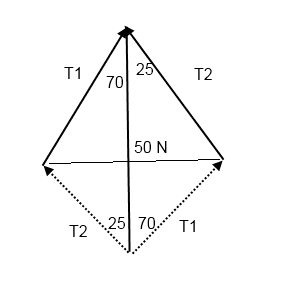# Vectors Tension Question

## Homework Statement

A 50 N sign is suspended by two cables that make angles of 25 degrees and 70 degrees with the horizontal. Determine the tension in each cable.

## Homework Equations

Cosine law, sine law, or trig

## The Attempt at a Solution

I believe that the teacher's answer is wrong. She did it the same way as the following person:I did it with the exact same diagram, same angles, but instead of using sine and cosine law, I just used normal trig and divided the tension in half for the triangle's vertical component, resulting in a different answer for the tensions.

This leads me to the conclusion that both answers are wrong, because you cannot just assume there is a 90 degree angle for where the tension hits the horizontal.

Is this assumption correct, and can I have a proper way to do this, with a proper answer?

#### Attachments

rock.freak667
Homework Helper
I did it with the exact same diagram, same angles, but instead of using sine and cosine law, I just used normal trig and divided the tension in half for the triangle's vertical component, resulting in a different answer for the tensions.

Due to the cables being at different angles, the tension in each one would be different. You assumed that T1 = 1/2T2.

This leads me to the conclusion that both answers are wrong, because you cannot just assume there is a 90 degree angle for where the tension hits the horizontal.

Is this assumption correct, and can I have a proper way to do this, with a proper answer?

From the triangle formed, it was assumed that the sign was straight when being held such that a perpendicular line was drawn from the vertex to the base.

I actually mean that the 50N downward force was divided into 2, so there would be a 25N force on each side for the vertical. This is based off of parallelogram properties.

rock.freak667
Homework Helper
I actually mean that the 50N downward force was divided into 2, so there would be a 25N force on each side for the vertical. This is based off of parallelogram properties.

The vertical components of the tension are not the same, so they do not take up the same amount of load.

Based on the diagram provided above, wouldn't they have to? Looking at the top triangle:

and looking at the bottom triangle:

Therefore they should be same, as based on the fact that parallelograms' diagonals bisect.

rock.freak667
Homework Helper
Based on the diagram provided above, wouldn't they have to? Looking at the top triangle:

and looking at the bottom triangle:

Therefore they should be same, as based on the fact that parallelograms' diagonals bisect.

I am not sure why the entire parallelogram was drawn since the actual forces are only one half so you'd really have the triangle. How it is drawn, it looks like the sign is supported by four cables and not two. They would be the same, but in the analysis, you doubled it all which lead to halving of the 50 N force.

Do you think you could please draw a proper diagram for me? I am not understanding what you are saying.

rock.freak667
Homework Helper
Last edited by a moderator:
So is it fair to say you cannot use the parallelogram method, because it would have a different vertical tension on each side of the parallelogram?

rock.freak667
Homework Helper
So is it fair to say you cannot use the parallelogram method, because it would have a different vertical tension on each side of the parallelogram?

No you can, it is reduced to the triangle in the same way. To get the forces such that the resultant of T1 and T2 is 50, you'd need to use either the left half or right half of your diagram (which is reduced to the same as mine).

rock.freak667
Homework Helper
My diagram is badly drawn for the intent of the parallelogram law, mine would be better suited for using equilibrium conditions. Nothing is to stop it from looking like yours, it is just that you'd need to redraw it better with the 50 N being vertical like in your original diagram.

In the analysis, you'd just choose one half of the parallelogram and do the analysis there.

Looking at it like this:Now I'm wondering if it's possible to have a 90 degree angle for the vertical, while still connect to make the parallelogram. Wouldn't the diagonal line represent the 50 N force, and the 2 lines going to the horizontal add up to be 50, but are different values?

#### Attachments

My main problem with understanding this, is shouldn't the line that I added to your picture:be perpendicular, resulting in 25N of force on each side?

I do not understand why you cannot do this.

#### Attachments

rock.freak667
Homework Helper
My main problem with understanding this, is shouldn't the line that I added to your picture:be perpendicular, resulting in 25N of force on each side?

I do not understand why you cannot do this.

I can't say if that line joining the two end points would be perpendicular. But you can draw from one end point to the vertical line to be 90 degrees.